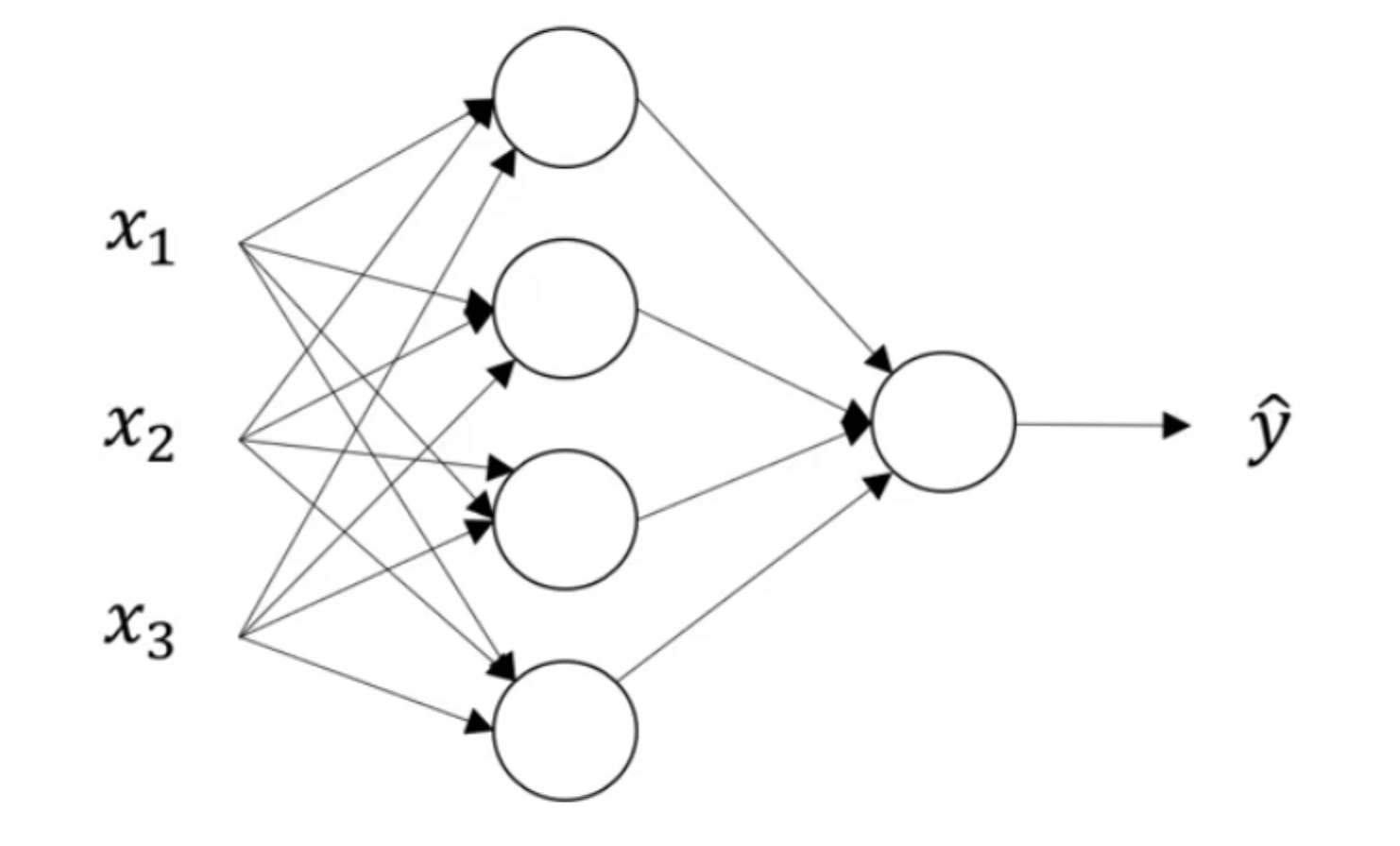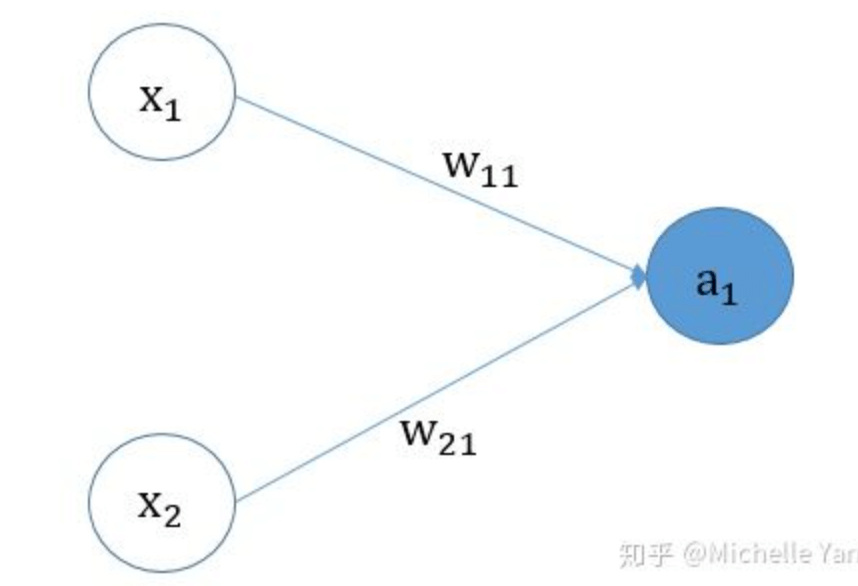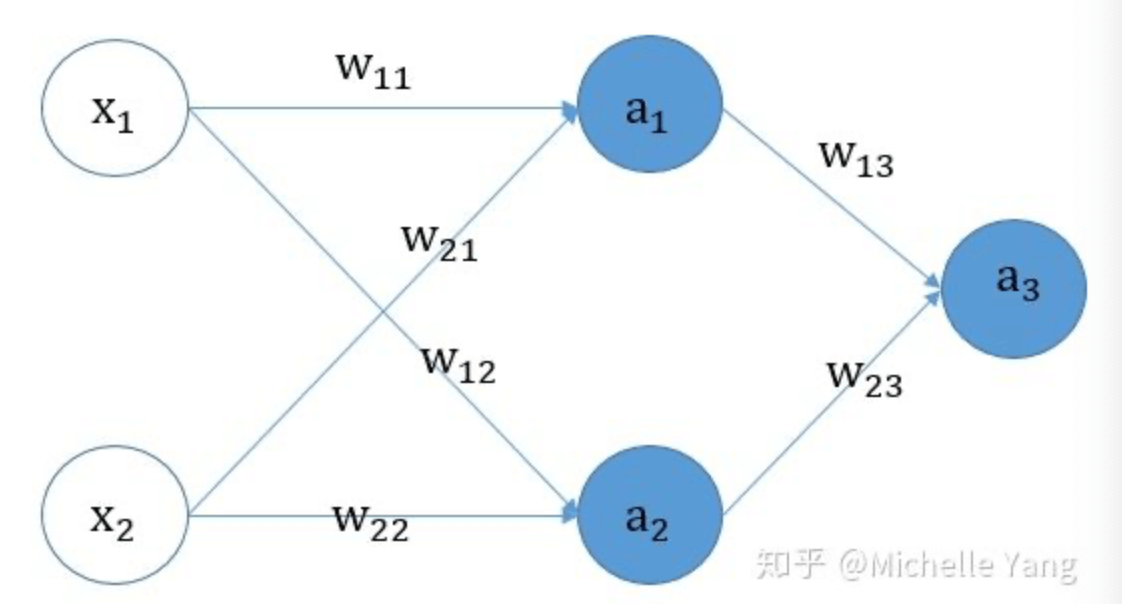# 【深度学习基础】第八课：神经网络的梯度下降法

## 神经网络的梯度下降法，网络参数的随机初始化

Posted by x-jeff on December 24, 2019

【深度学习基础】系列博客为学习Coursera上吴恩达深度学习课程所做的课程笔记。

# 1.神经网络的梯度下降法

【深度学习基础】第四课：正向传播与反向传播一文中我们了解了反向传播的原理，学习了梯度下降法在logistic回归中的应用。其实，logistic回归模型就可以看作是一个没有隐藏层的神经网络结构。那么，梯度下降法在一个带有隐藏层的浅层神经网络中是怎么应用的呢？这便是本文所要讨论的内容。## 1.1.单样本的情况

$x=a^{}=\begin{bmatrix} x_1 \\ x_2 \\ \vdots \\ x_m \\ \end{bmatrix} _{m \times 1}$

$w^{}=\begin{bmatrix} \cdots & {w^{}_1}^T & \cdots \\ \cdots & {w^{}_2}^T & \cdots \\ \cdots & {w^{}_3}^T & \cdots \\ \cdots & {w^{}_4}^T & \cdots \\ \end{bmatrix}_{4\times m}$ $b^{}=\begin{bmatrix} b^{}_1 \\ b^{}_2 \\ b^{}_3 \\ b^{}_4 \\ \end{bmatrix}_{4\times 1}$

$a^{}=\begin{bmatrix} a^{}_1 \\ a^{}_2 \\ a^{}_3 \\ a^{}_4 \\ \end{bmatrix}_{4\times 1}=\sigma (z^{})=\sigma (\begin{bmatrix} z^{}_1 \\ z^{}_2 \\ z^{}_3 \\ z^{}_4 \\ \end{bmatrix}_{4\times 1} )$

$w^{}=\begin{bmatrix} \cdots & {w^{}_1}^T & \cdots \\ \end{bmatrix}_{1\times 4}$ $b^{}=\begin{bmatrix} b^{}_1 \end{bmatrix}_{1\times 1}$

$a^{}=\begin{bmatrix} a^{}_1 \end{bmatrix}_{1\times 1}=\sigma (z^{})=\sigma (\begin{bmatrix} z^{}_1 \end{bmatrix}_{1\times 1})$

1. $da^{}=-\frac{y}{a^{}}+\frac{1-y}{1-a^{}}$；维数为$1\times 1$。
2. $dz^{}=a^{}-y$；维数为$1\times 1$。
3. $dw^{}=dz^{}{a^{}}^T$；维数为$1\times 4=(1\times 1)(1\times 4)$。
4. $db^{}=dz^{}$；维数为$1\times 1$。
5. $da^{}=dz^{}{w^{}}^T$；维数为$4\times 1$。
6. $dz^{}=da^{} * g^{’}(z^{})$；维数为$4\times 1$。*表示$da^{}$中的每个元素都乘上$g^{’}(z^{})$。其中，$g^{’}(z^{})=a^{}(1-a^{})$。
7. $dw^{}=dz^{}{a^{}}^T$；维度为$4\times m=(4\times 1)(1\times m)$。
8. $db^{}=dz^{}$；维度为$4\times 1$。

## 1.2.多个样本的情况

n个样本的情况和单样本基本类似：

1. $dZ^{}=A^{}-Y$；维度为$1\times n$。
2. $dw^{}=\frac{1}{n} dZ^{}{A^{}}^T$；维数为$1\times 4=(1\times n)(n \times 4)$。
3. $db^{}=\frac{1}{n} np.sum(dZ^{},axis=1,keepdims=True)$；维度为$1\times 1$。
4. $dZ^{}=dA^{}*g^{’}(Z^{})$；维数为$4\times n$。
5. $dw^{}=\frac{1}{n} dZ^{} {A^{}}^T$；维数为$4\times m=(4\times n)(n \times m)$。
6. $db^{}=\frac{1}{n} np.sum(dZ^{},axis=1,keepdims=True)$；维数为$4\times 1$。

# 2.网络参数的随机初始化

‼️如果将神经网络的各参数数组全部初始化为0，会使得梯度下降算法完全无效。

👉但是如果只是逻辑回归的话，可以将所有参数都初始化为0。• $a_1=sigmoid(w_{11}x_1+w_{21}x_2+b)$
• $L=-ylog(a_1)-(1-y)log(1-a_1)$

• $da_1=-\frac{y}{a_1}+\frac{1-y}{1-a_1}$
• $dw_{11}=(a_1-y)x_1$
• $dw_{21}=(a_1-y)x_2$
• $db=(a_1-y)$

👉现在考虑神经网络中的所有参数被初始化为0的情况。• $a_1=g(w_{11}x_1+w_{21}x_2+b_1)$
• $a_2=g(w_{12}x_1+w_{22}x_2+b_2)$
• $a_3=sigmoid(w_{13}a_1+w_{23}a_2+b_3)$
• $L=-ylog a_3-(1-y)log(1-a_3)$

• $da_3=-\frac{y}{a_3}+\frac{1-y}{1-a_3}$
• $dw_{13}=(a_3-y)a_1$
• $dw_{23}=(a_3-y)a_2$
• $db_3=(a_3-y)$
• $da_1=(a_3-y)w_{13}$
• $da_2=(a_3-y)w_{23}$
• $dw_{12}=da_2*a_2’*x_1$
• $dw_{22}=da_2*a_2’*x_2$
• $db_2=da_2*a_2’$
• $dw_{11}=da_1*a_1’*x_1$
• $dw_{21}=da_1*a_1’*x_2$
• $db_1=da_1*a_1’$

✓情况一：模型所有权重w初始化为0，所有偏置b初始化为0。

✓情况二：模型所有权重w初始化为0，所有偏置b随机初始化。

$w_{11},w_{12},w_{21},w_{22}$除了第一次迭代无法更新，后续迭代也可以正常更新。

$w^{}=np.random.randn((2,2))*0.01$

$b^{}=np.zero((2,1))$

# 4.参考资料